# Trees

## Trees

### Definitions:

Binary Tree:  A binary tree is either empty or it consists of a node called the root together with two binary trees called the left subtree and the right subtree of the root.

Node:  Each element of a binary tree is called a node of a tree.

Father:  If A is the root of the binary tree and B is the root of its left or right subtree, then A is said to be the father of B and B is said to be the left or right son of A.

Child:  A node is a child node if it originates from a parent node.

Leaf:  A node that has no sons is called a leaf.  A leaf node is also called external node.

Internal Node:  The nodes, which have child nodes are called internal or interior nodes.

External Path Length:  The external path length of a binary tree is the sum of all the levels of all the external nodes of its extension.

Internal Path Length:  The internal path length of a binary tree is the sum of all the levels of all the internal nodes of its extension.

Ancestor:  Node n1 is an ancestor of node n2, if n1 is either the father of n2 or the father of some ancestor of n2.

Descendant:  Node n2 is a descendant of n1, if n2 is the left or right son or exists at any other lower level than n1.

Left Descendant:  A node n2 is a left descendant of node n1 if n2 is either the left son of n1 or a descendant of the left son of n1.

Right Descendant:  A node n2 is a right descendant of node n1 if n2 is either the right son of n1 or a descendant of the right son of n1.

Strictly Binary Tree:  If every non-leaf node in a binary tree has non-empty left and right subtrees, the tree is termed as Strictly binary tree.  A Strictly binary tree with n leaves always contains (2n-1) nodes.

Level of a node:

The root of the tree has level 0 and the level of any other node in the tree is one more than the level of its father.  Example: In the following tree, the level of G is 3, of D is 2 and of root, A is 0.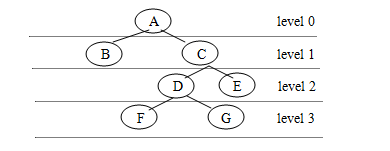Depth of  a Tree:

The depth of a binary tree is the maximum level of any leaf in the tree.  This equals the length of the longest path from the root to any leaf.  Thus the depth of the above binary tree is 3.

Complete Binary Tree:

A Complete binary tree of depth d is the Strictly binary tree of all of whose leaves are at level d (eg. if depth is 2), the complete binary tree is:NOTE:

1.  If a binary tree contains M nodes at level l, it contains at most 2m nodes at level l+1.

Example:  Let at level 1, number of nodes, m=2, then at level l+1 i.e. 1+1=2, nodes=2m=2*2=4.

2.  Since a binary tree can contain atmost one node at level 0 (the root), it can contain atmost one node 2L nodes at level L i.e. if level l is 3, then number of nodes = 2L =2 3 =8.  Thus at the most 8 nodes can be kept at level 3 in a CBT.

3.  A complete binary tree of depth d is the binary tree of depth d, that contains exactly 2L nodes at each level L, between 0 and d.

Let depth, d=2, L=2 {0,1,2}; nodes = {20,21,22} i.e. {1,2,4}.Almost Complete Binary Tree:

A Binary tree of depth d is an almost complete binary tree, if:

1. Any node nd at level less than (d-1) has two sons.
2. For any node nd in the tree, with a right descendant at level d, nd must have a left descendant/son and every left descendant of nd is either a leaf at level d or has two sons.(Numbering of nodes is done from left to right for the same level).

In the above example, depth =2

1.  node B at level 2-1 = 1 has 2 sons.

2.  At level 1, B has a right as well as a left descendant and C does not have left or right son.  Thus it is an example of an almost complete binary tree.

An almost complete strictly binary tree with n leaves has (2n-1) nodes as does any other strictly binary tree with n leaves.

An almost complete binary tree with n leaves that is not strictly binary has 2n nodes.
Binary Search Tree:

A binary search tree is a binary tree that is either empty or in which each node contains a key that satisfies the conditions:

1.  All keys (if any) in the left subtree of the root precede the key in the root.

2.  The key in the root precedes all keys (if any) in its right subtree.

3.  The left and right subtrees of the root are again search trees.

Orchards, Trees and Binary Trees:

Tree:  It is any set of points (called vertices) and any set of pairs of distinct vertices (called edges or branches) such that-

(1) There is a sequence of edges (a path) from any vertex to any other; and

(2) There are no circuits, that is, no paths starting from a vertex and returning to the same vertex.

Such trees may be termed as Free Trees.  But the trees that we study are almost always tied up by having one particular vertex singled out as the root and for emphasis we call such a tree as a Rooted Tree.

A rooted tree can be drawn in our usual way by picking it up by its root and shaking it so that all the branches and other vertices hand downward with the leaves at the bottom.  But there is no way to order nodes.

An Ordered Tree may be defined to be a rooted tree in which the children of each vertex are assigned an order.

2-Trees are rooted trees with the property that every vertex has either 0 or 2 children.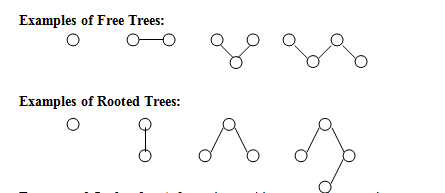Forests and Orchards:  A forest is an arbitrary set of trees, where trees are sorted trees.  An orchard set of ordered trees is called forest or orchard.

We can build a rooted or an ordered tree by starting with a forest or an orchard attaching a new vertex at the top and adding branches from the new vertex (which will the the root) to the roots of all trees in the forest or the orchard.

Conversion of General Trees to a Binary Tree:

To convert a general tree in to a binary tree, begin from root and join the nodes in left to right order for the same level that originate from the same root.  Remove all other links except left links and reconstruct the tree that will be a binary tree as follows:

Example: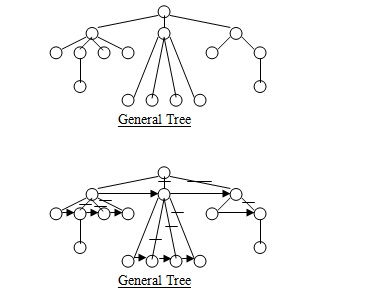Note: Arrow indicator shows the links to created for childs of same nodes at same level from left to right.  A hyphen (-) indicates the links to be deleted.

The binary tree for the above general tree is drawn below: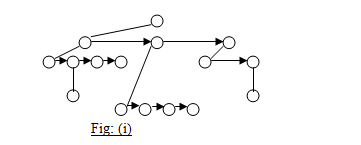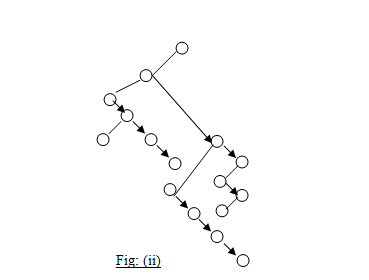Conversion of an Orchard/Forest to Binary Tree:

Follow the same rule as applicable for conversion of a general tree to a binary tree.  The second general tree becomes the right child of the root of the first tree and so on.  The resultant tree is binary tree equivalent of a given forest or orchard.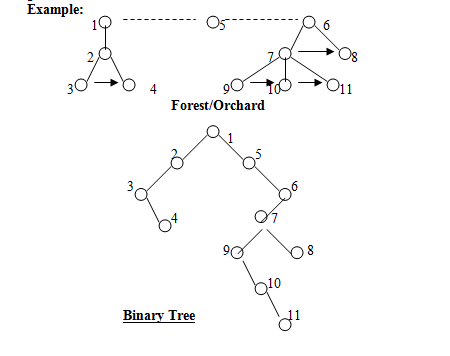Binary Tree Traversals:

Preorder (also known as Depth-first order)

• Visit the root.
• Traverse the left subtree in preorder.
• Traverse the right subtree in preorder.

Inorder (or Symmetric order)

• Traverse the left subtree in inorder.
• Visit the root.
• Traverse the right subtree in inorder.

Postorder (or End order)

• Traverse the left subtree in postorder.
• Traverse the right subtree in postorder.
• Visit the root.Expression Tree:

An expression tree is build up from the simple operands and operators of an arithmetical or logical expression by placing the simple operands as the leaves of a binary tree and the operators as the interior nodes.  For each binary operator, the left subtree contains all the simple operands and operators in the left operand of the left operand of the given operator and the right subtree contains everything in the right operand.

For a unary operator, one subtree will be empty.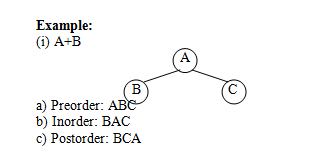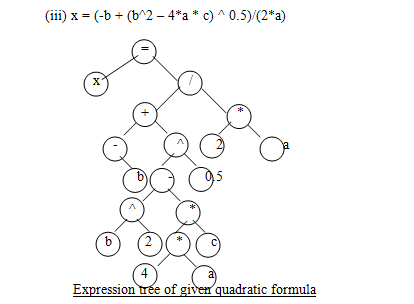Polish Notations: Traversal of expression trees results in polish forms of expressions-

1. Traversal of an expression tree in preorder yields the prefix form of the expression, in which every operator is written before its operands.

2.  Inorder traversal gives the infix form.

3.  Postorder traversal gives the postfix form, in which all operators appear after their operands.

Tree Sort:  Inorder traversal of a tree yields sorted output, if it is a Binary Search Tree.  We may simple take the items to be sorted, build them into a binary search tree and use inorder traversal to put them in order.